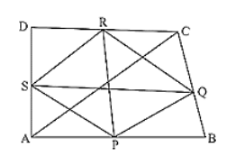# Show that, the line segments joining the mid-points of opposite sides of a quadrilateral bisects each other.

Question:

Show that, the line segments joining the mid-points of opposite sides of a quadrilateral bisects each other.

Solution:

Let ABCD is a quadrilateral in which P, Q, R and S are mid-points of sides AB, BC, CD and DA respectively.

So, by using mid-point theorem we can say that

SP ∥ BD and SP = (1/2) BD          .... (i)

Similarly in ΔBCD

QR ∥ BD and QR = (1/2) BD          .... (ii)

From equations (i) and (ii), we have

SP ∥ QR and SP = QR

As in quadrilateral SPQR, one pair of opposite sides is equal and parallel to each other.

So, SPQR is a parallelogram since the diagonals of a parallelogram bisect each other.

Hence PR and QS bisect each other.